Question

Draw the following schematic circuit diagram and label them accordingly:

(a) Draw the schematic for a circuit in which a 10 V battery, a 100 resistor, and a 220 resistor are all in series with one another. Determine the voltage across each resistor and the current owing through each resistor.
(b) Draw the schematic for a circuit in which a 10 V battery, a 100 resistor, and a 220 resistor are all in parallel with one another. Determine the voltage across each resistor and the current owing through each resistor.
(c) Draw the schematic for a circuit in which a 100 mA current source, a 100 resistor, and a 220 resistor are all in series with one another. Determine the voltage across each resistor and the current owing through each resistor. Use an appropriate schematic symbol for an ideal current source.
(h) Draw the schematic for a circuit in which a 100 mA current source, a 100 resistor, and a 220 resistor are all in parallel with one another. Determine the voltage across each resistor and the current owing through each resistor.

We need at least 10 more requests to produce the answer.

0 / 10 have requested this problem solution

The more requests, the faster the answer.

All students who have requested the answer will be notified once they are available.

#### Earn Coins

Coins can be redeemed for fabulous gifts.

Similar Homework Help Questions
• ### 8. Suppose the voltage output of a battery is 10.0V in a series circuit, and the...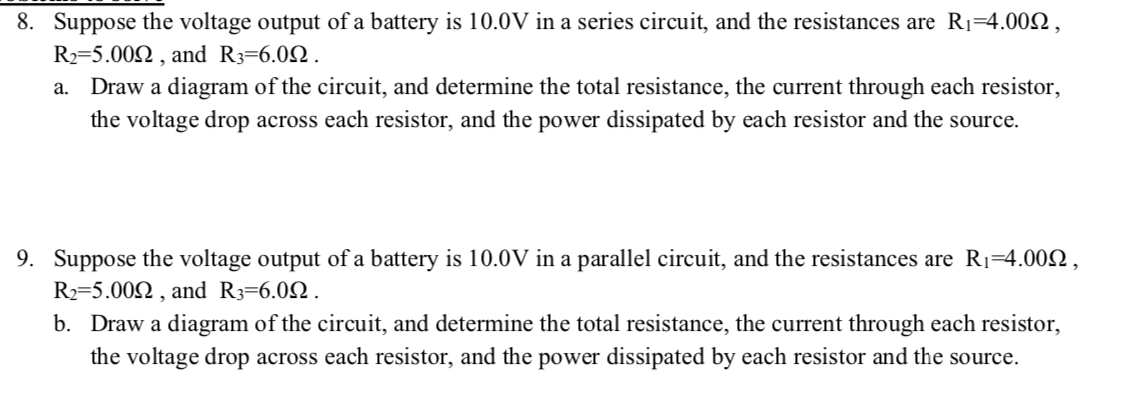8. Suppose the voltage output of a battery is 10.0V in a series circuit, and the resistances are Ri=4.0012, R2=5.0092 , and R3=6.092 . Draw a diagram of the circuit, and determine the total resistance, the current through each resistor, the voltage drop across each resistor, and the power dissipated by each resistor and the source. a. 9. Suppose the voltage output of a battery is 10.0V in a parallel circuit, and the resistances are Ri=4.0012, R2=5.0092 , and R3=6.092....

• ### 8. Suppose the voltage output of a battery is 10.0V in a series circuit, and the...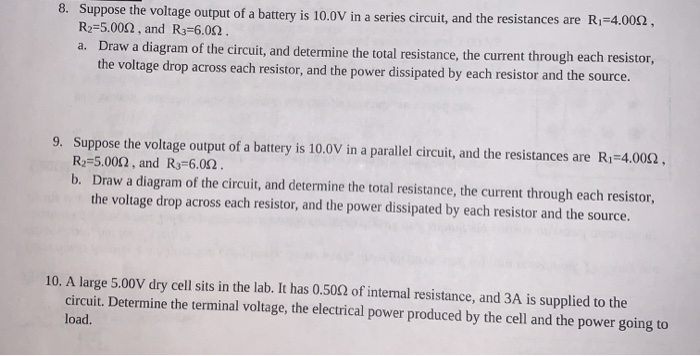8. Suppose the voltage output of a battery is 10.0V in a series circuit, and the resistances are Ri=4.0012, R2=5.0092, and R3=6.002. a. Draw a diagram of the circuit, and determine the total resistance, the current through each resistor, the voltage drop across each resistor, and the power dissipated by each resistor and the source. 9. Suppose the voltage output of a battery is 10.0V in a parallel circuit, and the resistances are Ri=4.0012, R=5.0002, and R3=6.092. b. Draw a...

• ### Part Five: Circuit Analysis 5 is now closed. Draw the schematic en find the voltage across...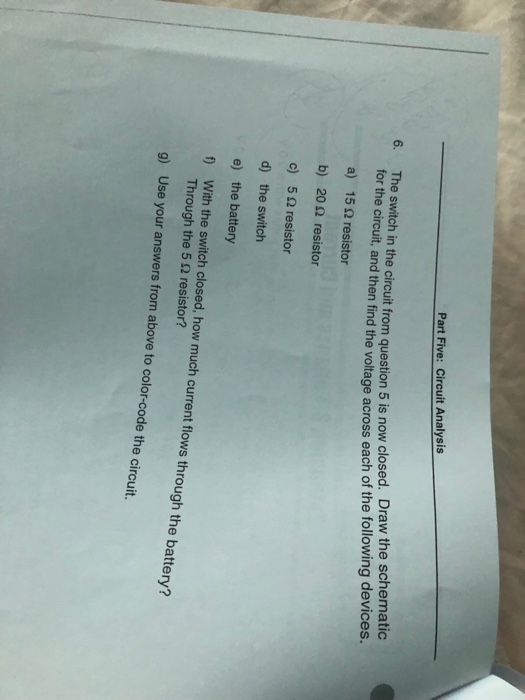Part Five: Circuit Analysis 5 is now closed. Draw the schematic en find the voltage across each of the following devices. 6. T he switch in the circuit from question for the circuit, and a) 15 Ω resistor b) 202 resistor c) 5 Ω resistor d) the switch e) the battery f With the switch closed, how much current flows through the batte th Through the 5 Ω resistor? Use your answers from above to color-code the circuit, g) 5....

• ### You have 20 and 80 (unit is the upside down U) resistors in a circuit with a 100 V battery

You have 20 and 80 (unit is the upside down U) resistors in a circuit with a 100 V battery. A)These resistors are parallel. Find the current through each resistor and the voltage drop across each resistor. B) These resistors are connected in series. Find the current through each resistor and the voltage drop across each resistor.

• ### 9. Suppose the voltage output of a battery is 10.0V in a parallel circuit, and the...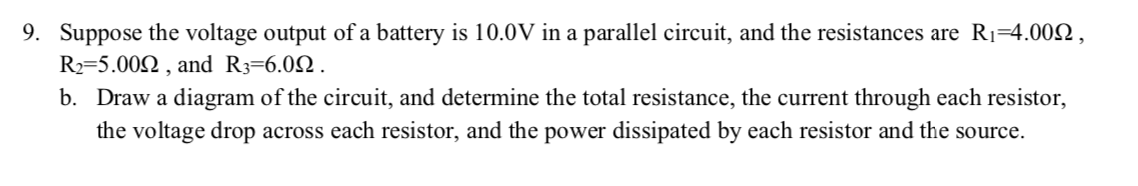9. Suppose the voltage output of a battery is 10.0V in a parallel circuit, and the resistances are Ri=4.0022, R2=5.0012 , and R3=6.01 . b. Draw a diagram of the circuit, and determine the total resistance, the current through each resistor, the voltage drop across each resistor, and the power dissipated by each resistor and the source.

• ### 9. Suppose the voltage output of a battery is 10.0V in a parallel circuit, and the...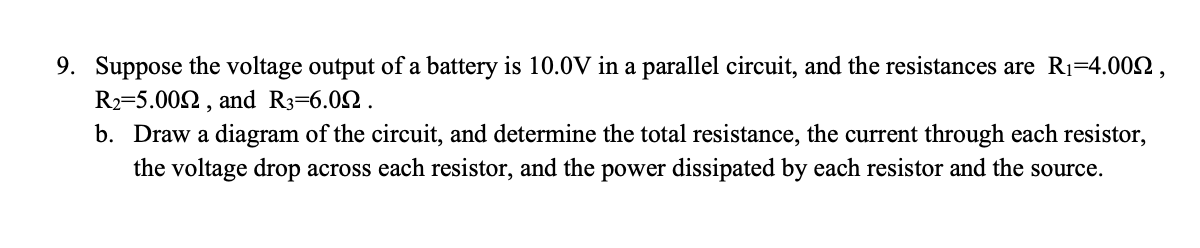9. Suppose the voltage output of a battery is 10.0V in a parallel circuit, and the resistances are Ri=4.0012, R2=5.0012, and R3=6.092. b. Draw a diagram of the circuit, and determine the total resistance, the current through each resistor, the voltage drop across each resistor, and the power dissipated by each resistor and the source.

• ### please draw the circuit diagram! thank you Part A (Draw the circuit diagram. Label all known...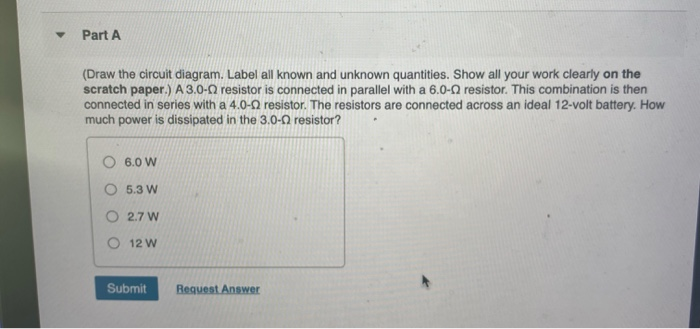please draw the circuit diagram! thank you Part A (Draw the circuit diagram. Label all known and unknown quantities. Show all your work clearly on the scratch paper.) A 3.0-0 resistor is connected in parallel with a 6.0-0 resistor. This combination is then connected in series with a 4.0-9 resistor. The resistors are connected across an ideal 12-volt battery. How much power is dissipated in the 3.0-9 resistor? 6.0 W O 5.3 W O 2.7 W O 12 W Submit...

• ### For the following questions, draw the circuit and solve for the unknown quantity requested. It is...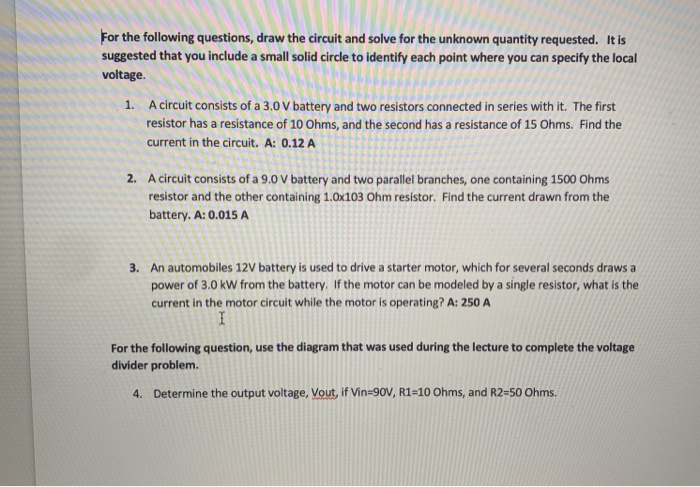For the following questions, draw the circuit and solve for the unknown quantity requested. It is suggested that you include a small solid circle to identify each point where you can specify the local voltage. voltage. 1. A circuit consists of a 3.0 V battery and two resistors connected in series with it. The first resistor has a resistance of 10 Ohms, and the second has a resistance of 15 Ohms. Find the current in the circuit. A: 0.12 A...

• ### The resistor should read 10.0 Ω. Tap on the battery so that the voltage adjustment window pops up. Adjust the voltage to 10.0 V.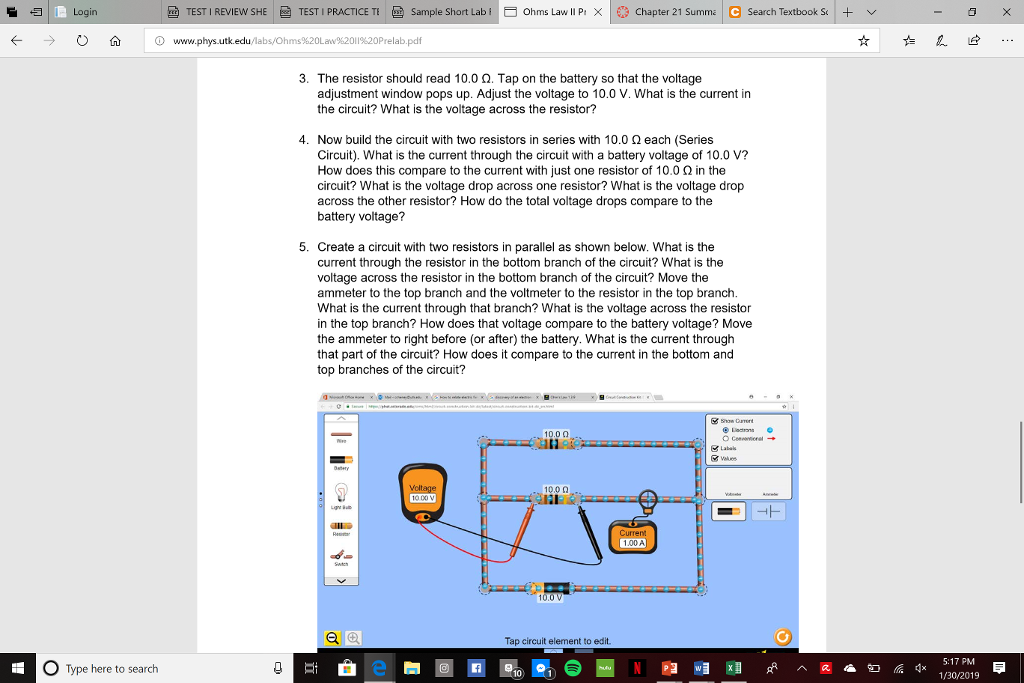3. The resistor should read 10.0 Ω. Tap on the battery so that the voltage adjustment window pops up. Adjust the voltage to 10.0 V. What is the current in the circuit? What is the voltage across the resistor? 4. Now build the circuit with two resistors in series with 10.0 2 each (Series Circuit). What is the current through the circuit with a battery voltage of 10.0 V? How does this compare to the current with just one resistor of...

• ### 2502 In the adjoining circuit schematic, in steady-state, the current flowing through the loop causes a...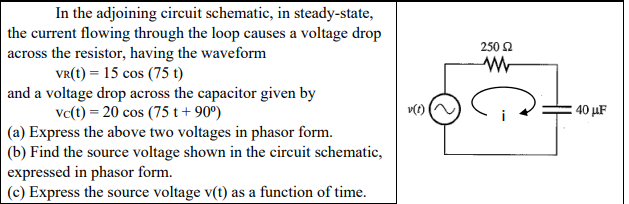2502 In the adjoining circuit schematic, in steady-state, the current flowing through the loop causes a voltage drop across the resistor, having the waveform vr(t) = 15 cos (75 t) and a voltage drop across the capacitor given by ve(t) = 20 cos (75 t +90°) (a) Express the above two voltages in phasor form. (b) Find the source voltage shown in the circuit schematic, expressed in phasor form. (c) Express the source voltage v(t) as a function of time....